# Arduino and HC-12 Long Range Wireless Communication Module

In this Arduino tutorial we will learn how to use the HC-12 wireless serial communication module which is capable of making a long range wireless communication between multiple Arduino boards, with distances up to 1.8km. You can watch the following video or read the written tutorial below for more details.

## Overview

For this tutorial I made two basic examples explaining the how to connect the HC-12 module and make a basic communication between two Arduinos and an additional example where using an accelerometer sensor at the first Arduino I wirelessly control the position of the stepper at the second Arduino.[/column]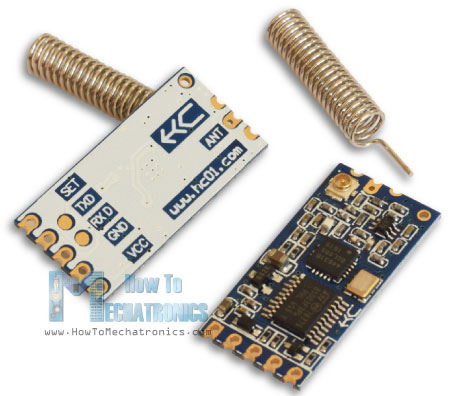## HC-12 Wireless Communication Module

First let’s take a closer look at the HC-12 wireless serial port communication module. Here are some specification:

• Its wireless working frequency band is from 433.4 MHz to 473.0 MHz
• It has a total of 100 channels with a stepping of 400 KHz between each channel
• Transmitting power is from -1dBm (0.79mW) to 20dBm (100mW)
• Receiving sensitivity is from -117dBm (0.019pW) to -100dBm (10pW).

These values actually depend on the selected Serial and Over-the-Air Baud Rate as seen in the table.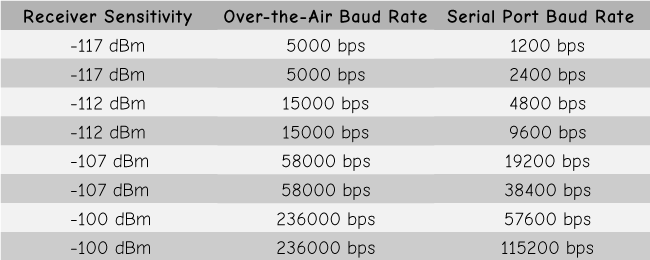The HC-12 module has a microcontroller which actually doesn’t have to be programmed by the user. For configuring the module we simply use AT commands, which can be sent from an Arduino, a PC, or any other microcontroller using the serial port. For entering the AT command mode we just have to set the “Set” pin of the module to a low logic level.

## Arduino and HC-12

Now let’s connect the HC-12 module to the Arduino and make the first example. Here’s the circuit schematics. The operating voltage of the module is from 3.2 V to 5.5 V and for more stable work it is recommended to use a decoupling capacitor and an external power supply. However, I used the PC USB as power for all three examples in this tutorial and didn’t have any problem with it.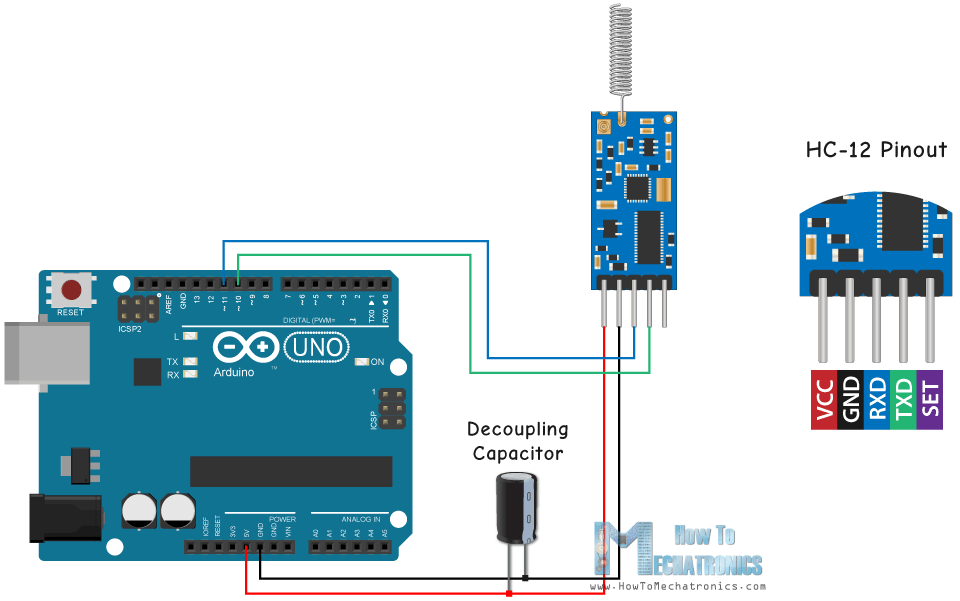I connected the first module to an Arduino UNO and the second module to an Arduino MEGA, but of course, you can use any board you want.

You can get the components needed for this Arduino Tutorial from the links below:

Disclosure: These are affiliate links. As an Amazon Associate I earn from qualifying purchases.

## Example 01 – Arduino Code

Here’s the Arduino code for the first example, a basic communication between the two modules using the Serial Monitor.

```/*    Arduino Long Range Wireless Communication using HC-12
Example 01
by Dejan Nedelkovski, www.HowToMechatronics.com
*/

#include <SoftwareSerial.h>

SoftwareSerial HC12(10, 11); // HC-12 TX Pin, HC-12 RX Pin

void setup() {
Serial.begin(9600);             // Serial port to computer
HC12.begin(9600);               // Serial port to HC12

}

void loop() {
while (HC12.available()) {        // If HC-12 has data
Serial.write(HC12.read());      // Send the data to Serial monitor
}
while (Serial.available()) {      // If Serial monitor has data
HC12.write(Serial.read());      // Send that data to HC-12
}
}```

The same code is used for both Arduinos. We can connect the two Arduinos on two separate computers but also we can use a single computer.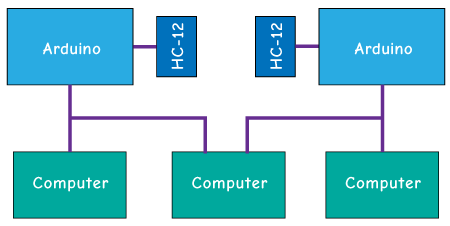In that case, once we connect the first Arduino to the computer, we need to select the model and the COM port and upload the code to the Arduino. Then we connect the second Arduino and we have to start the Arduino IDE again in order to be able to select the other COM port to which our second Arduino is connected, and then upload the same code.

So once we have the two Arduino IDEs running we can start the serial monitors and test whether the communication works properly. Anything we type in the serial monitor will be sent from one to the other Arduino.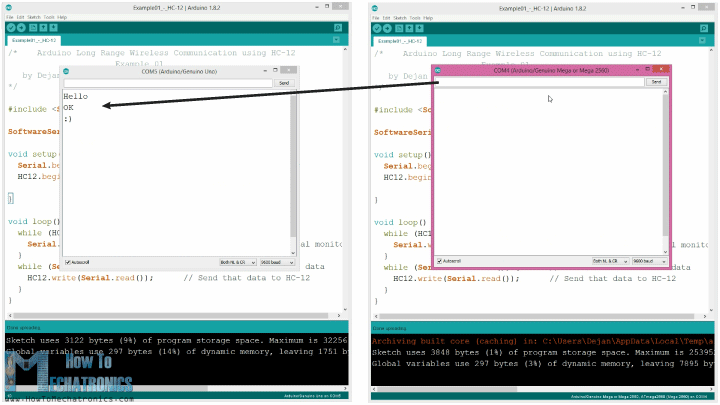How the code works: So once we type something in the serial monitor and click the Send button, at the first Arduino, the while loop with the Serial.available() function will become true and using the HC12.write() function we will send the data from the serial monitor to the HC-12 module. This module will transfer the data wirelessly to the second HC-12 module, so at the second Arduino the while loop with the HC12.available() function will become true and using the Serial.write() function the data will be sent to the serial monitor.

We can use the same code for sending AT Commands and configuring the module parameters. All we have to do is connect the “Set” pin of the module to Ground or any digital pin of the Arduino and set the pin to low logic level.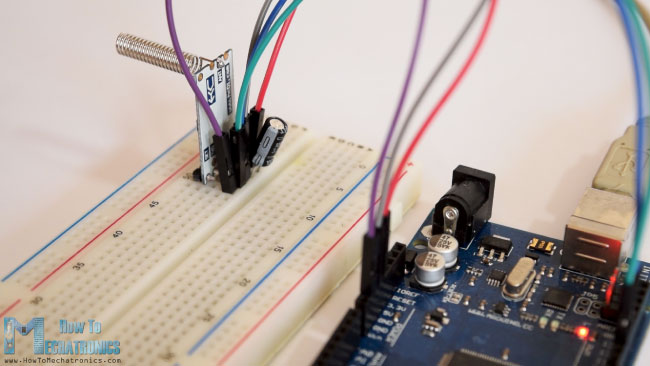To test whether we have successfully enter the mode, in the serial monitor we can type “AT” and we should get a response message “OK”. There are total of 12 AT Commands, and they are used for changing various parameters like the baud rate, the channel, the transmitting power etc.  For example, if we type “AT+B38400” the baud rate of the module will be set to 38400.

### AT Commands:

1. AT – Test command.

Example: Send “AT” to module, and the module returns “OK”.

2. AT+Bxxxx – Change the serial port baud rate.

Available baud rates: 1200 bps, 2400 bps, 4800 bps, 9600 bps, 19200 bps, 38400 bps, 57600 bps, and 115200 bps. Default: 9600 bps.

Example: Send “AT+B38400” to module, and the module returns “OK+B19200”.

3. AT+Cxxxx – Change wireless communication channel, from 001 to 100.

Default: Channel 001, with working frequency of 433.4MHz. Each next channel is 400KHz higher.

Example: If we want to set the module to channel 006, we need to send “AT+C006” command to the module, and the module will return “OK+C006”. The new working frequency will be 435.4MHz.

## Example 02

Now let’s move the second example. Here we will use two push buttons for selecting different communication channels and see a different method of storing the incoming data.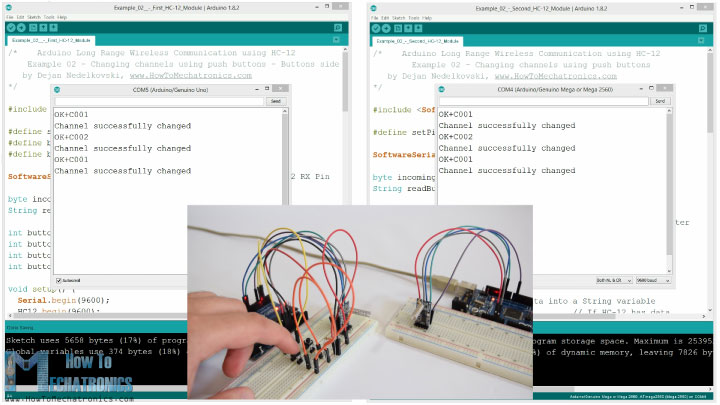Note: The “Set” pins of both HC-12 modules are connected to the pins number 6 of the two Arduinos and the two buttons, at the first Arduino, to the pins 4 and 3.

First Arduino code:

```/*    Arduino Long Range Wireless Communication using HC-12
Example 02 - Changing channels using push buttons - Buttons side
by Dejan Nedelkovski, www.HowToMechatronics.com
*/

#include <SoftwareSerial.h>

#define setPin 6
#define button1 4
#define button2 3

SoftwareSerial HC12(10, 11);         // HC-12 TX Pin, HC-12 RX Pin

byte incomingByte;

int button1State = 0;
int button1Pressed = 0;
int button2State = 0;
int button2Pressed = 0;

void setup() {
Serial.begin(9600);                   // Open serial port to computer
HC12.begin(9600);                     // Open serial port to HC12
pinMode(setPin, OUTPUT);
pinMode(button1, INPUT);
pinMode(button2, INPUT);
digitalWrite(setPin, HIGH);           // HC-12 normal, transparent mode
}

void loop() {
// ==== Storing the incoming data into a String variable
while (HC12.available()) {             // If HC-12 has data
incomingByte = HC12.read();          // Store each icoming byte from HC-12
}
delay(100);
// ==== Sending data from one HC-12 to another via the Serial Monitor
while (Serial.available()) {
}

// ==== If button 1 is pressed, set the channel 01
if (button1State == HIGH & button1Pressed == LOW) {
button1Pressed = HIGH;
delay(20);
}
if (button1Pressed == HIGH) {
HC12.print("AT+C001");               // Send the AT Command to the other module
delay(100);
//Set AT Command Mode
digitalWrite(setPin, LOW);           // Set HC-12 into AT Command mode
delay(100);                          // Wait for the HC-12 to enter AT Command mode
HC12.print("AT+C001");               // Send AT Command to HC-12
delay(200);
while (HC12.available()) {           // If HC-12 has data (the AT Command response)
Serial.write(HC12.read());         // Send the data to Serial monitor
}
Serial.println("Channel successfully changed");
digitalWrite(setPin, HIGH);          // Exit AT Command mode
button1Pressed = LOW;
}

// ====  If button 2 is pressed, set the channel 02
if (button2State == HIGH & button2Pressed == LOW) {
button2Pressed = HIGH;
delay(100);
}
if (button2Pressed == HIGH) {
HC12.print("AT+C002"); // Send the AT Command to the other module
delay(100);
//Set AT Command Mode
digitalWrite(setPin, LOW);           // Set HC-12 into AT Command mode
delay(100);                          // Wait for the HC-12 to enter AT Command mode
HC12.print("AT+C002");               // Send AT Command to HC-12
delay(200);
while (HC12.available()) {           // If HC-12 has data (the AT Command response)
Serial.write(HC12.read());         // Send the data to Serial monitor
}
Serial.println("Channel successfully changed");
digitalWrite(setPin, HIGH);
button2Pressed = LOW;
}
checkATCommand();
}
// ==== Custom function - Check whether we have received an AT Command via the Serial Monitor
void checkATCommand () {
if (readBuffer.startsWith("AT")) {     // Check whether the String starts with "AT"
digitalWrite(setPin, LOW);           // Set HC-12 into AT Command mode
delay(200);                          // Wait for the HC-12 to enter AT Command mode
HC12.print(readBuffer);              // Send AT Command to HC-12
delay(200);
while (HC12.available()) {           // If HC-12 has data (the AT Command response)
Serial.write(HC12.read());         // Send the data to Serial monitor
}
digitalWrite(setPin, HIGH);          // Exit AT Command mode
}
}```

Second Arduino code:

```/*    Arduino Long Range Wireless Communication using HC-12
Example 02 - Changing channels using push buttons
by Dejan Nedelkovski, www.HowToMechatronics.com
*/

#include <SoftwareSerial.h>

#define setPin 6

SoftwareSerial HC12(10, 11); // HC-12 TX Pin, HC-12 RX Pin

byte incomingByte;

void setup() {
Serial.begin(9600);             // Open serial port to computer
HC12.begin(9600);               // Open serial port to HC12
pinMode(setPin, OUTPUT);
digitalWrite(setPin, HIGH);     // HC-12 normal mode
}

void loop() {
// ==== Storing the incoming data into a String variable
while (HC12.available()) {             // If HC-12 has data
incomingByte = HC12.read();          // Store each icoming byte from HC-12
}
delay(100);
// ==== Sending data from one HC-12 to another via the Serial Monitor
while (Serial.available()) {
}
// === If button 1 is pressed, set channel 01
digitalWrite(setPin, LOW);           // Set HC-12 into AT Command mode
delay(100);                          // Wait for the HC-12 to enter AT Command mode
HC12.print(readBuffer);              // Send AT Command to HC-12 ("AT+C001")
delay(200);
while (HC12.available()) {           // If HC-12 has data (the AT Command response)
Serial.write(HC12.read());         // Send the data to Serial monitor
}
Serial.println("Channel successfully changed");
digitalWrite(setPin, HIGH);          // Exit AT Command mode
}
// === If button 2 is pressed, set channel 02
digitalWrite(setPin, LOW);           // Set HC-12 into AT Command mode
delay(100);                          // Wait for the HC-12 to enter AT Command mode
HC12.print(readBuffer);              // Send AT Command to HC-12
delay(200);
while (HC12.available()) {           // If HC-12 has data (the AT Command response)
Serial.write(HC12.read());         // Send the data to Serial monitor
}
Serial.println("Channel successfully changed");
digitalWrite(setPin, HIGH);          // Exit AT Command mode

}
checkATCommand();
}
// ==== Custom function - Check whether we have received an AT Command via the Serial Monitor
void checkATCommand () {
if (readBuffer.startsWith("AT")) {     // Check whether the String starts with "AT"
digitalWrite(setPin, LOW);           // Set HC-12 into AT Command mode
delay(100);                          // Wait for the HC-12 to enter AT Command mode
HC12.print(readBuffer);              // Send AT Command to HC-12
delay(200);
while (HC12.available()) {           // If HC-12 has data (the AT Command response)
Serial.write(HC12.read());         // Send the data to Serial monitor
}
digitalWrite(setPin, HIGH);          // Exit AT Command mode
}
}```

Description of the codes:

So, first we need to define the pins and set the “Set” pin to high logic level in order the module to work in normal, transparent mode. With the first while loop we store the incoming data into a String variable, so we can better handle it.

```// ==== Storing the incoming data into a String variable
while (HC12.available()) {             // If HC-12 has data
incomingByte = HC12.read();          // Store each icoming byte from HC-12
}```

The incoming data always comes one byte at a time, so for example if we send the string “Test123” from second Arduino, this while loop will do 7 iterations. Each iteration, using the HC12.read() function we will read each incoming byte or character and add it to the String variable named “readBuffer”.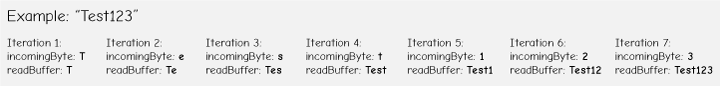Next let’s see how we can change the communication channel using the first push button. So if we press the first push button, using the HC12.print() function we will send the string “AT+C001”  to the HC-12 module or to the second Arduino.

```if (button1Pressed == HIGH) {
HC12.print("AT+C001");               // Send the AT Command to the other module
delay(100);
//Set AT Command Mode
digitalWrite(setPin, LOW);           // Set HC-12 into AT Command mode
delay(100);                          // Wait for the HC-12 to enter AT Command mode
HC12.print("AT+C001");               // Send AT Command to HC-12
delay(200);
while (HC12.available()) {           // If HC-12 has data (the AT Command response)
Serial.write(HC12.read());         // Send the data to Serial monitor
}
Serial.println("Channel successfully changed");
digitalWrite(setPin, HIGH);          // Exit AT Command mode
button1Pressed = LOW;
}```

When this string will be received at the second Arduino, we will set the HC-12 module into AT command mode, and then write the same string “AT+C001” to it which will set the module to communication channel number one.

```// At the second Arduino

// === If button 1 is pressed, set channel 01
digitalWrite(setPin, LOW);           // Set HC-12 into AT Command mode
delay(100);                          // Wait for the HC-12 to enter AT Command mode
HC12.print(readBuffer);              // Send AT Command to HC-12 ("AT+C001")
delay(200);
while (HC12.available()) {           // If HC-12 has data (the AT Command response)
Serial.write(HC12.read());         // Send the data to Serial monitor
}
Serial.println("Channel successfully changed");
digitalWrite(setPin, HIGH);          // Exit AT Command mode
}```

We use the next while loop to print the response message from the HC-12 module whether the channel has been successfully changed.

```while (HC12.available()) {           // If HC-12 has data (the AT Command response)
Serial.write(HC12.read());         // Send the data to Serial monitor
}```

Back at the first Arduino, we do the same procedure of sending the AT command to the first HC-12 module. In the same way we, using the pushing the second button, we set the communication channel number two. So using this method we can select, at any time, with which HC-12 module we will communicate.

At the end, the checkATCommand() custom function, checks whether the received message is an AT command, by checking whether the string starts with “AT”. If so, the module enters the AT command mode and executes the command.

```// ==== Custom function - Check whether we have received an AT Command via the Serial Monitor
void checkATCommand () {
if (readBuffer.startsWith("AT")) {     // Check whether the String starts with "AT"
digitalWrite(setPin, LOW);           // Set HC-12 into AT Command mode
delay(200);                          // Wait for the HC-12 to enter AT Command mode
HC12.print(readBuffer);              // Send AT Command to HC-12
delay(200);
while (HC12.available()) {           // If HC-12 has data (the AT Command response)
Serial.write(HC12.read());         // Send the data to Serial monitor
}
digitalWrite(setPin, HIGH);          // Exit AT Command mode
}
}```

## HC-12 Wireless Communication: Stepper Motor Control using an Accelerometer

Now let’s take a look at the third example. Here we control the position of the stepper motor at the second Arduino, using the accelerometer module at the first Arduino.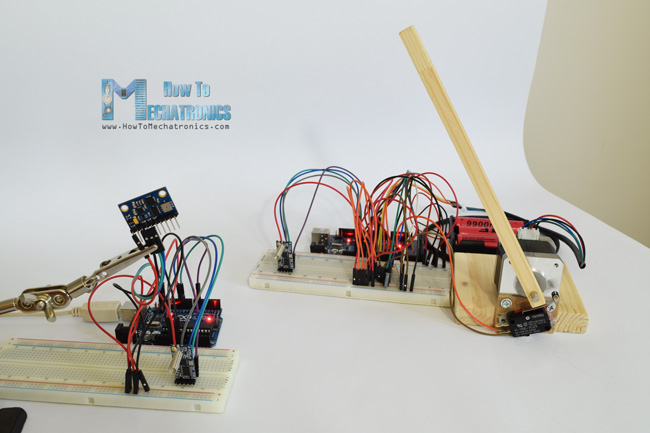The circuit also contains a microswitch for finding the initial position of the stepper motor at 0 degrees.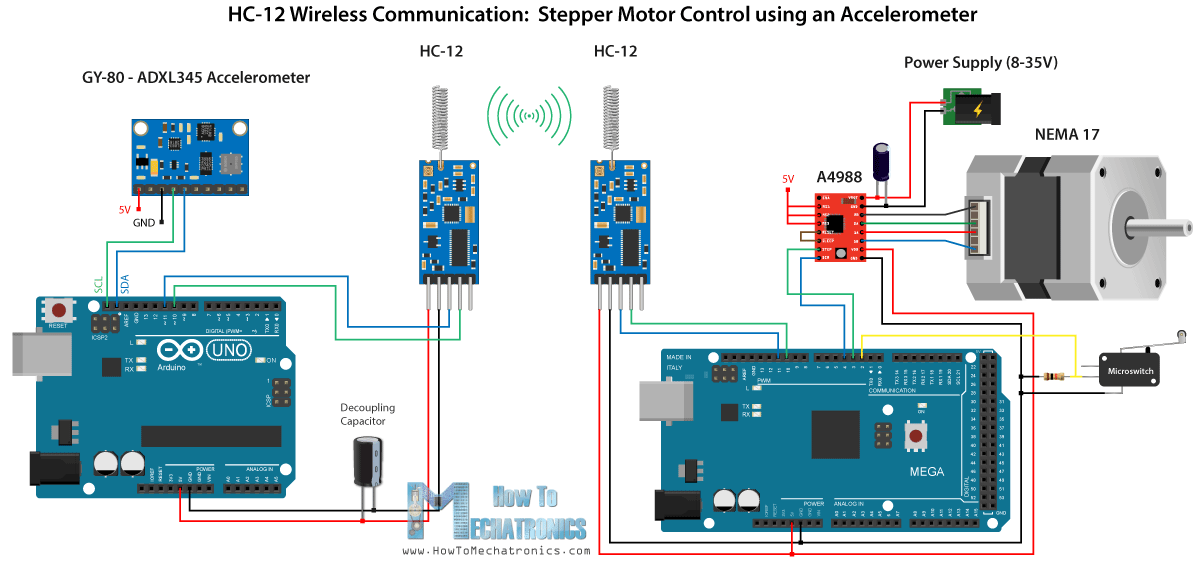You can get the components needed for this example from the links below:

Disclosure: These are affiliate links. As an Amazon Associate I earn from qualifying purchases.

Note here that I already have detailed tutorials on how to connect and use both the accelerometer and the stepper motor, so for this example I will only explain the HC-12 part of the code.

First Arduino – Transmitter code:

```/*   Arduino Long Range Wireless Communication using HC-12
Example 03 - Stepper Motor Control using Accelerometer - Transmitter, Accelerometer
by Dejan Nedelkovski, www.HowToMechatronics.com
*/

#include <SoftwareSerial.h>
#include <Wire.h>

SoftwareSerial HC12(10, 11); // HC-12 TX Pin, HC-12 RX Pin

float angle;
int lastAngle = 0;
int count = 0;
int angleSum = 0;

#define Power_Register 0x2D
#define Y_Axis_Register_DATAY0 0x34
#define Y_Axis_Register_DATAY1 0x35
#define Z_Axis_Register_DATAZ0 0x36
#define Z_Axis_Register_DATAZ1 0x37
int X0, X1, X_out;
int Y0, Y1, Y_out;
int Z1, Z0, Z_out;
float Xa, Ya, Za;

void setup() {
HC12.begin(9600);               // Open serial port to HC12
Wire.begin(); // Initiate the Wire library
Serial.begin(9600);
delay(100);

Wire.write(Power_Register); // Power_CTL Register
// Enable measurement
Wire.write(8); // Bit D3 High for measuring enable (0000 1000)
Wire.endTransmission();
}
void loop() {
// X-axis
//Ask the particular registers for data
Wire.write(X_Axis_Register_DATAX0);
Wire.write(X_Axis_Register_DATAX1);
Wire.endTransmission(); // Ends the transmission and transmits the data from the two registers
Wire.requestFrom(ADXAddress, 2); // Request the transmitted two bytes from the two registers
if (Wire.available() <= 2) { //
/* Converting the raw data of the X-Axis into X-Axis Acceleration
- The output data is Two's complement
- X0 as the least significant byte
- X1 as the most significant byte */
X1 = X1 << 8;
X_out = X0 + X1;
Xa = X_out / 256.0; // Xa = output value from -1 to +1, Gravity acceleration acting on the X-Axis
}
//Serial.print("Xa= ");
//Serial.println(X_out);

// Y-Axis
Wire.write(Y_Axis_Register_DATAY0);
Wire.write(Y_Axis_Register_DATAY1);
Wire.endTransmission();
if (Wire.available() <= 2) {
Y1 = Y1 << 8;
Y_out = Y0 + Y1;
Ya = Y_out / 256.0;
}

// Combine X and Y values for getting the angle value from 0 to 180 degrees
if (Y_out > 0) {
angle = map(Y_out, 0, 256, 90, 0);
}
else if (Y_out < 0) {
angle = map(Y_out, 256, 0, 90, 0);
angle = 90 - angle;
}
if (X_out < 0 & Y_out < 0) {
angle = 180;
}
if (X_out < 0 & Y_out >0) {
angle = 0;
}

// float to int
int angleInt = int(angle);
// Makes 100 accelerometer readings and sends the average for smoother result
angleSum = angleSum + angleInt;
count++;
if (count >= 100) {
angleInt = angleSum / 100;
angleSum = 0;
count = 0;
// Some more smoothing of acceleromter reading - sends the new angle only if it differes from the previous one by +-2
if (angleInt > lastAngle + 2 || angleInt < lastAngle - 2) {
Serial.println(angleInt);
String angleString = String(angleInt);
//sends the angle value with start marker "s" and end marker "e"
HC12.print("s" + angleString + "e");
delay(10);
lastAngle = angleInt;
angleSum = 0;
count = 0;
}
}
}
```

```/*   Arduino Long Range Wireless Communication using HC-12
Example 03 - Stepper Motor Control using Accelerometer - Receiver, Stepper Motor

by Dejan Nedelkovski, www.HowToMechatronics.com
*/
#include <SoftwareSerial.h>

SoftwareSerial HC12(10, 11); // HC-12 TX Pin, HC-12 RX Pin

char incomingByte;

// defines pins numbers
const int dirPin = 4;
const int stepPin = 3;
const int button = 2;

int currentAngle = 0;
int lastAngle = 0;
int rotate = 0;

void setup() {
Serial.begin(9600);             // Open serial port to computer
HC12.begin(9600);               // Open serial port to HC12

// Sets the two pins as Outputs
pinMode(dirPin, OUTPUT);
pinMode(stepPin, OUTPUT);
// Microswitch input, with internal pull-up resistor activated
pinMode(button, INPUT_PULLUP);
delay(10);
digitalWrite(dirPin, HIGH);
boolean startingPosition = true;
while (startingPosition) {
digitalWrite(stepPin, HIGH);
delayMicroseconds(200);
digitalWrite(stepPin, LOW);
delayMicroseconds(200);
startingPosition = false;
}
}
delay(100);
}
void loop() {
boolean start = false;
while (HC12.available()) {             // If HC-12 has data
incomingByte = HC12.read();          // Store each icoming byte from HC-12
delay(5);
// Reads the data between the start "s" and end marker "e"
if (start == true) {
if (incomingByte != 'e') {
}
else {
start = false;
}
}
// Checks whether the received message statrs with the start marker "s"
else if ( incomingByte == 's') {
start = true; // If true start reading the message
}
}
// Converts the string into integer
// Makes sure it uses angles between 0 and 180
if (currentAngle > 0 && currentAngle < 180) {
// Convert angle value to steps (depending on the selected step resolution)
// A cycle = 200 steps, 180deg = 100 steps ; Resolution: Sixteenth step x16
currentAngle = map(currentAngle, 0, 180, 0, 1600);
//Serial.println(currentAngle); // Prints the angle on the serial monitor
digitalWrite(dirPin, LOW); // Enables the motor to move in a particular direction
// Rotates the motor the amount of steps that differs from the previous positon
if (currentAngle != lastAngle) {
if (currentAngle > lastAngle) {
rotate = currentAngle - lastAngle;
for (int x = 0; x < rotate; x++) {
digitalWrite(stepPin, HIGH);
delayMicroseconds(400);
digitalWrite(stepPin, LOW);
delayMicroseconds(400);
}
}
// rotate the other way
if (currentAngle < lastAngle) {
rotate = lastAngle - currentAngle;
digitalWrite(dirPin, HIGH);        //Changes the rotations direction
for (int x = 0; x < rotate; x++) {
digitalWrite(stepPin, HIGH);
delayMicroseconds(400);
digitalWrite(stepPin, LOW);
delayMicroseconds(400);
}
}
}
lastAngle = currentAngle;  // Remembers the current/ last positon
}
}```

Description of the codes:

So first we defining the pins and initializing the modules in the setup section. Then we read the values of the X and Y axis of the accelerometer and map them to a values from 0 to 180 degrees. The values coming from the accelerometer can sometimes be unstable or shake, so for smoothing the result I used the average value of one hundred readings.

```// Makes 100 accelerometer readings and sends the average for smoother result
angleSum = angleSum + angleInt;
count++;
if (count >= 100) {
angleInt = angleSum / 100;
angleSum = 0;
count = 0;
// Some more smoothing of acceleromter reading - sends the new angle only if it differes from the previous one by +-2
if (angleInt > lastAngle + 2 || angleInt < lastAngle - 2) {
Serial.println(angleInt);
String angleString = String(angleInt);
//sends the angle value with start marker "s" and end marker "e"
HC12.print("s" + angleString + "e");
delay(10);
lastAngle = angleInt;
angleSum = 0;
count = 0;
}
}```

For even further smoothing I will send the new value of the angle only if it differs from the previous by 2.

Note here that when sending the angle to the HC-12 module, I’m also sending the character “s” in front, and the character “e” after, which will help me when receiving the data at the second Arduino.

At the second Arduino we wait until the start marker “s” comes, then we read the value of the angle until the end marker “e” arrive. This way we are sure that we will receive only the value of the angle.

```// Reads the incoming angle
while (HC12.available()) {             // If HC-12 has data
incomingByte = HC12.read();          // Store each icoming byte from HC-12
delay(5);
// Reads the data between the start "s" and end marker "e"
if (start == true) {
if (incomingByte != 'e') {
}
else {
start = false;
}
}
// Checks whether the received message statrs with the start marker "s"
else if ( incomingByte == 's') {
start = true; // If true start reading the message
}
}```

Then we convert the value to integer, and map the value from 0 to 1600 steps, which corresponds to the selected sixteenth step resolution at the A4988 stepper driver. Then we rotate the stepper motor to the current angle.

So that would be all for this Arduino tutorial. Feel free to ask any question in the comments section below.

### 14 thoughts on “Arduino and HC-12 Long Range Wireless Communication Module”

1.Hello sir, can you please tell me the max range that can be achieved.Can I make a remote from this module to control a plane

•The maximum range is 1.8 kilometers in open space.

2.I think this is a terrific project, and I’d like to use something similar to remotely control a solenoid. I do have two questions, though:

1. You mention a “decoupling capacitor,” but not its value or orientation. Can you explain this in a little more detail?

2. Is there any danger of someone else interfering with the signal to grab control of the receiving arduino? Basically, is tampering a worry?

•The decoupling capacitor provides stability and smooths the power supply to the module. As for the value you can use anything from 0.1 to 10uF. Negative terminal goes to ground and positive to VCC.
As for tampering, I don’t have much info on that but I don’t believe that this cheap module can provide high security.

3.Hello Dejan … where can I buy … the HC-12 module..??
I have scanned the NET… found none..!!!
have they stopped it..??? (it maybe a security risk… as in south africa.. 433 mHz code-hopping has been … hi-jacked… I know I was a victim)..
(contact me.. I’ll tell you how I BEAT the crooks!)..
thanks..!!

4.Could you send the information directly from the arduino to a laptop or pc wirelessly?

•Well yes, it’s possible, but still you need two Arduinos. One sends the data, the other is accepts the data and gives it to the PC via the serial port.

•Yes its possible even with a single arduino but you have to use hc05 bt module (which will not give such a high range as hc12)

5.Dear Dejan,
nice tutorial, thank you. I have a question, does it possible to control two dc motors and one LED only with 1 link? I mean can I remotely control the motion of two dc motors and turning on or off a LED by this method?

•Hey, thanks! Well yes, you should be able to do that. You just have to send appropriate indicators and/ or values to the receiver. Of course, the code or the programming also depends on the control method of the dc motors.

6.wowwww nice tutorial indeed.
I have a question though, is there any way one can control the board my a mobile phone?. I mean can I control dc motors from my phone through Arduino hc-12?

•Hey thanks! For controlling the board with your mobile phone you will need to use a Bluetooth, like the HC-05 Bluetooth module.

7.hello,
nice tutorial!
I tried to make the same project but i have a problem with the range.
the connection is lost at 10 meters. We are far the 1.8 km announced by the datasheet. I tried to change the parameters but it does change anything.
What range do you have with your system? 10m, 100m, 500m?
Do you have any tip to help me?
•